# RD Sharma solutions for Mathematics for Class 9 chapter 12 - Congruent Triangles [Latest edition]

#### Chapters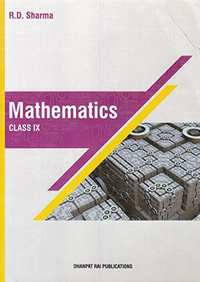## Solutions for Chapter 12: Congruent Triangles

Below listed, you can find solutions for Chapter 12 of CBSE RD Sharma for Mathematics for Class 9.

Exercise 12.1Exercise 12.2Exercise 12.3Exercise 12.4Exercise 12.5Exercise 12.6Exercise 12.7Exercise 12.8
Exercise 12.1 [Pages 15 - 16]

### RD Sharma solutions for Mathematics for Class 9 Chapter 12 Congruent Triangles Exercise 12.1 [Pages 15 - 16]

Exercise 12.1 | Q 1 | Page 15

In Fig. 10.22, the sides BA and CA have been produced such that: BA = AD and CA = AE.
Prove that segment DE || BC.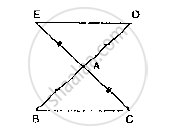Exercise 12.1 | Q 2 | Page 15

In a ΔPQR, if PQ = QR and L, M and N are the mid-points of the sides PQ, QR and RP
respectively. Prove that: LN = MN.

Exercise 12.1 | Q 3 | Page 15

Prove that the medians of an equilateral triangle are equal.

Exercise 12.1 | Q 4 | Page 15

In a ΔABC, if ∠A=l20° and AB = AC. Find ∠B and ∠C.

Exercise 12.1 | Q 5 | Page 15

In a ΔABC, if AB = AC and ∠B = 70°, find ∠A.

Exercise 12.1 | Q 6 | Page 15

The vertical angle of an isosceles triangle is 100°. Find its base angles.

Exercise 12.1 | Q 7 | Page 15

In Figure AB = AC and ∠ACD =105°, find ∠BAC.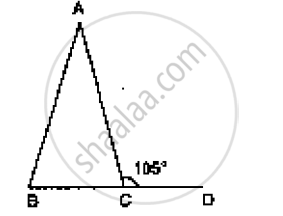Exercise 12.1 | Q 8 | Page 15

Find the measure of each exterior angle of an equilateral triangle.

Exercise 12.1 | Q 9 | Page 15

If the base of an isosceles triangle is produced on both sides, prove that the exterior angles so formed are equal to each other.

Exercise 12.1 | Q 10 | Page 15

In figure, AB = AC and DB = DC, find the ratio ∠ABD : ∠ACD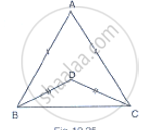Exercise 12.1 | Q 11 | Page 16

Determine the measure of each of the equal angles of a right-angled isosceles triangle.

Exercise 12.1 | Q 12 | Page 16

In Fig. 10.23, PQRS is a square and SRT is an equilateral triangle. Prove that
(i) PT = QT (ii) ∠TQR = 15°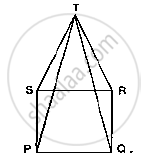Exercise 12.1 | Q 13 | Page 16

AB is a line seg P and Q are points on opposite sides of AB such that each of them is equidistant from the points A and B (See Fig. 10.26). Show that the line PQ is perpendicular bisector of AB.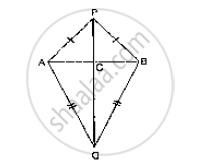Exercise 12.2 [Page 25]

### RD Sharma solutions for Mathematics for Class 9 Chapter 12 Congruent Triangles Exercise 12.2 [Page 25]

Exercise 12.2 | Q 1 | Page 25

BD and CE are bisectors of ∠B and ∠C of an isosceles ΔABC with AB = AC. Prove that BD = CE.

Exercise 12.2 | Q 2 | Page 25

In Fig. 10.40, it is given that RT = TS, ∠1 = 2∠2 and ∠4 = 2∠3. Prove that ΔRBT ≅ ΔSAT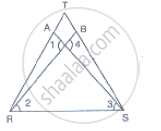Exercise 12.2 | Q 3 | Page 25

Two lines AB and CD intersect at O such that BC is equal and parallel to AD. Prove that the lines AB and CD bisect at O.

Exercise 12.3 [Page 47]

### RD Sharma solutions for Mathematics for Class 9 Chapter 12 Congruent Triangles Exercise 12.3 [Page 47]

Exercise 12.3 | Q 1 | Page 47

In two right triangles one side an acute angle of one are equal to the corresponding side and angle of the othe Prove that the triangles are congruent.

Exercise 12.3 | Q 2 | Page 47

If the bisector of the exterior vertical angle of a triangle be parallel to the base. Show that the triangle is isosce

Exercise 12.3 | Q 3 | Page 47

In an isosceles triangle, if the vertex angle is twice the sum of the base angles, calculate the angles of the triangle.

Exercise 12.3 | Q 4 | Page 47

Prove that each angle of an equilateral triangle is 60°.

Exercise 12.3 | Q 5 | Page 47

Angles A, B, C of a triangle ABC are equal to each other. Prove that ΔABC is equilateral.

Exercise 12.3 | Q 6 | Page 47

ABC is a right angled triangle in which ∠A = 90° and AB = AC. Find ∠B and ∠C.

Exercise 12.3 | Q 7 | Page 47

PQR is a triangle in which PQ = PR and S is any point on the side PQ. Through S, a line is drawn parallel to QR and intersecting PR at T. Prove that PS = PT.

Exercise 12.3 | Q 8 | Page 47

In a ΔABC, it is given that AB = AC and the bisectors of ∠B and ∠C intersect at O. If M is a point on BO produced, prove that ∠MOC = ∠ABC.

Exercise 12.3 | Q 9 | Page 47

P is a point on the bisector of an angle ∠ABC. If the line through P parallel to AB meets BC at Q, prove that triangle BPQ is isosceles.

Exercise 12.3 | Q 10 | Page 47

ABC is a triangle in which ∠B = 2 ∠C. D is a point on BC such that AD bisects ∠BAC and AB = CD.
Prove that ∠BAC = 72°.

Exercise 12.4 [Page 57]

### RD Sharma solutions for Mathematics for Class 9 Chapter 12 Congruent Triangles Exercise 12.4 [Page 57]

Exercise 12.4 | Q 1 | Page 57

In Fig. 10.92, it is given that AB = CD and AD = BC. Prove that ΔADC ≅ ΔCBA.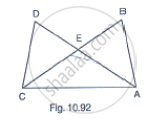Exercise 12.5 [Pages 61 - 62]

### RD Sharma solutions for Mathematics for Class 9 Chapter 12 Congruent Triangles Exercise 12.5 [Pages 61 - 62]

Exercise 12.5 | Q 1 | Page 61

ABC is a triangle and D is the mid-point of BC. The perpendiculars from D to AB and AC are equal. Prove that the triangle is isosceles.

Exercise 12.5 | Q 2 | Page 61

ABC is a triangle in which BE and CF are, respectively, the perpendiculars to the sides AC and AB. If BE = CF, prove that ΔABC is isosceles

Exercise 12.5 | Q 3 | Page 61

If perpendiculars from any point within an angle on its arms are congruent, prove that it lies on the bisector of that angle.

Exercise 12.5 | Q 4 | Page 61

In Fig. 10.99, AD ⊥ CD and CB ⊥. CD. If AQ = BP and DP = CQ, prove that ∠DAQ = ∠CBP.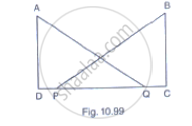Exercise 12.5 | Q 5.1 | Page 62

Which of the following statements are true (T) and which are false (F):

Sides opposite to equal angles of a triangle may be unequal

Exercise 12.5 | Q 5.2 | Page 62

Which of the following statements are true (T) and which are false (F):

Angles opposite to equal sides of a triangle are equal

Exercise 12.5 | Q 5.3 | Page 62

Which of the following statements are true (T) and which are false (F):

The measure of each angle of an equilateral triangle is 60°

Exercise 12.5 | Q 5.4 | Page 62

Which of the following statements are true (T) and which are false (F) :

If the altitude from one vertex of a triangle bisects the opposite side, then the triangle may be isosceles.

Exercise 12.5 | Q 5.5 | Page 62

Which of the following statements are true (T) and which are false (F):

The bisectors of two equal angles of a triangle are equal

Exercise 12.5 | Q 5.6 | Page 62

Which of the following statements are true (T) and which are false (F):

If the bisector of the vertical angle of a triangle bisects the base, then the triangle may be isosceles.

Exercise 12.5 | Q 5.7 | Page 62

Which of the following statements are true (T) and which are false (F):

The two altitudes corresponding to two equal sides of a triangle need not be equal.

Exercise 12.5 | Q 5.8 | Page 62

Which of the following statements are true (T) and which are false (F):

If any two sides of a right triangle are respectively equal to two sides of other right triangle, then the two triangles are congruent.

• True

• False

Exercise 12.5 | Q 5.9 | Page 62

Which of the following statements are true (T) and which are false (F):

Two right triangles are congruent if hypotenuse and a side of one triangle are respectively equal equal to the hypotenuse and a side of the other triangle.

• True

• False

Exercise 12.5 | Q 6.1 | Page 62

Fill the blank in the following so that the following statement is true.

Sides opposite to equal angles of a triangle are ......

Exercise 12.5 | Q 6.2 | Page 62

Fill the blank in the following so that the following statement is true.

Angle opposite to equal sides of a triangle are .....

Exercise 12.5 | Q 6.3 | Page 62

Fill the blank in the following so that the following statement is true.

In an equilateral triangle all angles are .....

Exercise 12.5 | Q 6.4 | Page 62

Fill the blank in the following so that the following statement is true.

In a ΔABC if ∠A = ∠C , then AB = ......

Exercise 12.5 | Q 6.5 | Page 62

Fill the blank in the following so that the following statement is true.

If altitudes CE and BF of a triangle ABC are equal, then AB = ....

Exercise 12.5 | Q 6.6 | Page 62

Fill the blank in the following so that the following statement is true.

In an isosceles triangle ABC with AB = AC, if BD and CE are its altitudes, then BD is …… CE.

Exercise 12.5 | Q 6.7 | Page 62

Fill the blank in the following so that the following statement is true.

In right triangles ABC and DEF, if hypotenuse AB = EF and side AC = DE, then ΔABC ≅ Δ ……

Exercise 12.5 | Q 7 | Page 62

ABCD is a square, X and Yare points on sides AD and BC respectively such that AY = BX. Prove that BY = AX and ∠BAY = ∠ABX.

Exercise 12.6 [Pages 81 - 82]

### RD Sharma solutions for Mathematics for Class 9 Chapter 12 Congruent Triangles Exercise 12.6 [Pages 81 - 82]

Exercise 12.6 | Q 1 | Page 81

In ΔABC, if ∠A = 40° and ∠B = 60°. Determine the longest and shortest sides of the triangle.

Exercise 12.6 | Q 2 | Page 81

In a ΔABC, if ∠B = ∠C = 45°, which is the longest side?

Exercise 12.6 | Q 3 | Page 81

In ΔABC, side AB is produced to D so that BD = BC. If ∠B = 60° and ∠A = 70°, prove that: (i) AD > CD (ii) AD > AC

Exercise 12.6 | Q 4 | Page 81

Is it possible to draw a triangle with sides of length 2 cm, 3 cm and 7 cm?

Exercise 12.6 | Q 5 | Page 81

In Δ ABC, ∠B = 35°, ∠C = 65° and the bisector of ∠BAC meets BC in P. Arrange AP, BP and CP in descending order.

Exercise 12.6 | Q 6 | Page 81

Prove that the perimeter of a triangle is greater than the sum of its altitudes.

Exercise 12.6 | Q 7.1 | Page 81

In the given figure, prove that:

CD + DA + AB + BC > 2AC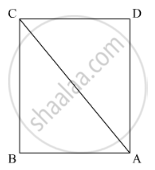Exercise 12.6 | Q 7.2 | Page 81

In the given figure, prove that:

CD + DA + AB > BCExercise 12.6 | Q 8.1 | Page 81

Which of the following statements are true (T) and which are false (F)?

Sum of the three sides of a triangle is less than the sum of its three altitudes.

Exercise 12.6 | Q 8.2 | Page 81

Which of the following statements are true (T) and which are false (F)?

Sum of any two sides of a triangle is greater than twice the median drawn to the third side.

Exercise 12.6 | Q 8.3 | Page 81

Which of the following statements are true (T) and which are false (F)?

Sum of any two sides of a triangle is greater than the third side.

Exercise 12.6 | Q 8.4 | Page 81

Which of the following statements are true (T) and which are false (F)?

Difference of any two sides of a triangle is equal to the third side.

Exercise 12.6 | Q 8.5 | Page 81

Which of the following statements are true (T) and which are false (F)?

If two angles of a triangle are unequal, then the greater angle has the larger side opposite to it.

Exercise 12.6 | Q 8.6 | Page 81

Which of the following statements are true (T) and which are false (F)?

Of all the line segments that can be drawn from a point to a line not containing it, the perpendicular line segment is the shortest one.

Exercise 12.6 | Q 9.1 | Page 82

Fill in the blank to make the following statement true.

In a right triangle the hypotenuse is the .... side.

Exercise 12.6 | Q 9.2 | Page 82

Fill in the blank to make the following statement true.

The sum of three altitudes of a triangle is ..... than its perimeter.

Exercise 12.6 | Q 9.3 | Page 82

Fill in the blank to make the following statement true.

The sum of any two sides of a triangle is .... than the third side.

Exercise 12.6 | Q 9.4 | Page 82

Fill in the blank to make the following statement true.

If two angles of a triangle are unequal, then the smaller angle has the........ side opposite to it.

Exercise 12.6 | Q 9.5 | Page 82

Fill in the blank to make the following statement true.

Difference of any two sides of a triangle is........ than the third side.

Exercise 12.6 | Q 9.6 | Page 82

Fill in the blank to make the following statement true.

If two sides of a triangle are unequal, then the larger side has .... angle opposite to it.

Exercise 12.6 | Q 10 | Page 82

O is any point in the interior of ΔABC. Prove that
(i) AB + AC > OB + OC
(ii) AB + BC + CA > OA + QB + OC
(iii) OA + OB + OC > 1/2(AB + BC + CA)

Exercise 12.6 | Q 11 | Page 82

Prove that in a quadrilateral the sum of all the sides is greater than the sum of its diagonals.

Exercise 12.7 [Page 84]

### RD Sharma solutions for Mathematics for Class 9 Chapter 12 Congruent Triangles Exercise 12.7 [Page 84]

Exercise 12.7 | Q 1 | Page 84

In two congruent triangles ABC and DEF, if AB = DE and BC = EF. Name the pairs of equal angles.

Exercise 12.7 | Q 2 | Page 84

In two triangles ABC and DEF, it is given that ∠A = ∠D, ∠B = ∠E and ∠C =∠F. Are the two triangles necessarily congruent?

Exercise 12.7 | Q 3 | Page 84

If ABC and DEF are two triangles such that AC = 2.5 cm, BC = 5 cm, ∠C = 75°, DE = 2.5 cm, DF = 5cm and ∠D = 75°. Are two triangles congruent?

Exercise 12.7 | Q 4 | Page 84

In two triangles ABC and ADC, if AB = AD and BC = CD. Are they congruent?

Exercise 12.7 | Q 5 | Page 84

In triangles ABC and CDE, if AC = CE, BC = CD, ∠A = 60°, ∠C = 30° and ∠D = 90°. Are two triangles congruent?

Exercise 12.7 | Q 6 | Page 84

ABC is an isosceles triangle in which AB = AC. BE and CF are its two medians. Show that BE = CF.

Exercise 12.7 | Q 7 | Page 84

Find the measure of each angle of an equilateral triangle.

Exercise 12.7 | Q 8 | Page 84

CDE is an equilateral triangle formed on a side CD of a square ABCD. Show that ΔADE ΔBCE.

Exercise 12.7 | Q 9 | Page 84

Prove that the sum of three altitudes of a triangle is less than the sum of its sides.

Exercise 12.7 | Q 10 | Page 84

In the given figure, if AB = AC and ∠B = ∠C. Prove that BQ = CP.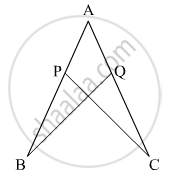Exercise 12.8 [Pages 85 - 88]

### RD Sharma solutions for Mathematics for Class 9 Chapter 12 Congruent Triangles Exercise 12.8 [Pages 85 - 88]

Exercise 12.8 | Q 1 | Page 85

Mark the correct alternative in each of the following:

If ABC ≅  ΔLKM, then side of ΔLKM equal to side AC of ΔABC is

• LK

• KM

• LM

•  None of these

Exercise 12.8 | Q 2 | Page 85

If ΔABC ≅  ΔABC is isosceles with

• AB = AC

• AB = BC

• AC = BC

• None of these

Exercise 12.8 | Q 3 | Page 85

ΔPQR and ΔABC is not congruent to ΔRPQ, then which of the following is not true:

• BC = PQ

• AC = PR

• AB = PQ

•  QR = BC

Exercise 12.8 | Q 4 | Page 85

In triangles ABC and PQR three equality relations between some parts are as follows:
AB = QP, ∠B = ∠P and BC = PR

State which of the congruence conditions applies:

•  SAS

• ASA

• SSS

• RHS

Exercise 12.8 | Q 5 | Page 85

In triangles ABC and PQR, if ∠A = ∠R, ∠B = ∠P and AB = RP, then which one of the following congruence conditions applies:

•  SAS

• ASA

• SSS

• RHS

Exercise 12.8 | Q 6 | Page 85

In ΔPQR ≅ ΔEFD then ED =

• PQ

• RQ

• PR

• None of these

Exercise 12.8 | Q 7 | Page 85

If ΔPQR≅ ΔEFD, then ∠E =

•  ∠P

•  ∠Q

•  ∠R

• None of these

Exercise 12.8 | Q 8 | Page 85

In a ΔABC, if AB = AC and BC is produced to D such that ∠ACD = 100°, then ∠A =

• 20°

• 40°

• 60°

• 80°

Exercise 12.8 | Q 9 | Page 85

In an isosceles triangle, if the vertex angle is twice the sum of the base angles, then the measure of vertex angle of the triangle is

•  100°

•  120°

•  110°

•  130°

Exercise 12.8 | Q 10 | Page 85

Which of the following is not a criterion for congruence of triangles?

• SAS

• SSA

• ASA

• SSS

Exercise 12.8 | Q 11 | Page 86

In the given figure, the measure of ∠B'A'C' is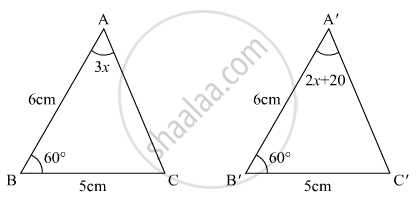•  50°

•  60°

•  70°

•  80°

Exercise 12.8 | Q 12 | Page 86

If ABC and DEF are two triangles such that ΔABC $\cong$ ΔFDE and AB = 5cm, ∠B = 40°

• DF = 5cm, ∠F = 60°

• DE = 5cm, ∠E = 60°

• DF = 5cm, ∠E = 60°

• DE = 5cm, ∠D = 40°

Exercise 12.8 | Q 13 | Page 86

In the given figure, AB ⊥ BE and FE ⊥ BE. If BC = DE and AB = EF, then ΔABD is congruent to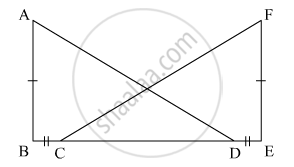• ΔEFC

• ΔECF

•  ΔCEF

•  ΔFEC

Exercise 12.8 | Q 14 | Page 86

In the given figure, if AE || DC and AB = AC, the value of ∠ABD is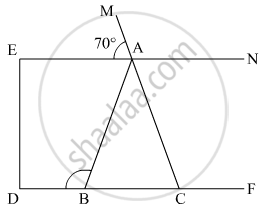• 70°

• 110°

• 120°

• 130°

Exercise 12.8 | Q 15 | Page 87

In the given figure, ABC is an isosceles triangle whose side AC is produced to E. Through C, CD is drawn parallel to BA. The value of x is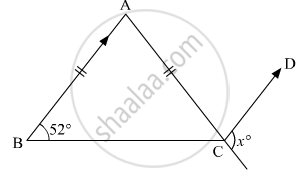• 52°

• 76°

• 156°

• 104°

Exercise 12.8 | Q 16 | Page 87

In the given figure, if AC is bisector of ∠BAD such that AB = 3 cm and AC =  5 cm, then CD =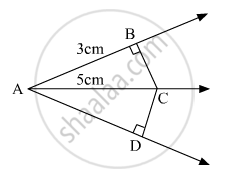•  2 cm

•  3 cm

•  4 cm

•  5 cm

Exercise 12.8 | Q 17 | Page 87

D, E, F are the mid-point of the sides BC, CA and AB respectively of ΔABC. Then ΔDEF is congruent to triangle

• ABC

• AEF

• BFD, CDE

•  AFE, BFD, CDE

Exercise 12.8 | Q 18 | Page 87

ABC is an isosceles triangle such that AB = AC and AD is the median to base BC. Then, ∠BAD =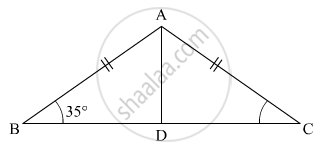•  55°

•  70°

• 35°

• 110°

Exercise 12.8 | Q 19 | Page 87

In the given figure, X is a point in the interior of square ABCD. AXYZ is also a square. If DY = 3 cm and AZ = 2 cm, then BY =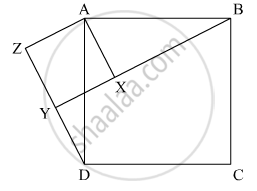• 5 cm

• 6 cm

• 7 cm

• 8 cm

Exercise 12.8 | Q 20 | Page 88

In the given figure, ABC is a triangle in which ∠B = 2∠C. D is a point on side BC such that ADbisects ∠BAC and AB = CD. BE is the bisector of ∠B. The measure of ∠BAC is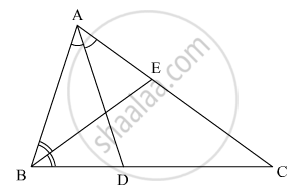• 72°

• 73°

• 74°

• 95°

## Solutions for Chapter 12: Congruent Triangles

Exercise 12.1Exercise 12.2Exercise 12.3Exercise 12.4Exercise 12.5Exercise 12.6Exercise 12.7Exercise 12.8## RD Sharma solutions for Mathematics for Class 9 chapter 12 - Congruent Triangles

Shaalaa.com has the CBSE Mathematics Mathematics for Class 9 CBSE solutions in a manner that help students grasp basic concepts better and faster. The detailed, step-by-step solutions will help you understand the concepts better and clarify any confusion. RD Sharma solutions for Mathematics Mathematics for Class 9 CBSE 12 (Congruent Triangles) include all questions with answers and detailed explanations. This will clear students' doubts about questions and improve their application skills while preparing for board exams.

Further, we at Shaalaa.com provide such solutions so students can prepare for written exams. RD Sharma textbook solutions can be a core help for self-study and provide excellent self-help guidance for students.

Concepts covered in Mathematics for Class 9 chapter 12 Congruent Triangles are Concept of Triangles - Sides, Angles, Vertices, Interior and Exterior of Triangle, Properties of a Triangle, Some More Criteria for Congruence of Triangles, Inequalities in a Triangle, Criteria for Congruence of Triangles, Congruence of Triangles.

Using RD Sharma Mathematics for Class 9 solutions Congruent Triangles exercise by students is an easy way to prepare for the exams, as they involve solutions arranged chapter-wise and also page-wise. The questions involved in RD Sharma Solutions are essential questions that can be asked in the final exam. Maximum CBSE Mathematics for Class 9 students prefer RD Sharma Textbook Solutions to score more in exams.

Get the free view of Chapter 12, Congruent Triangles Mathematics for Class 9 additional questions for Mathematics Mathematics for Class 9 CBSE, and you can use Shaalaa.com to keep it handy for your exam preparation.

Share